# Control Systems Design

This page will describe in detail the development of several different control strategies. These control systems are developed based on the quadrotor model derived in the System Modeling section, implemented in a simulated environment, and finally verified on an actual system. Lower level control systems will run at a high rate and control the quadrotor’s attitude. These controllers rely on the onboard IMU measurements. At a higher level, it is also possible to control the translational position of the quadrotor. Depending on the sensor used, this can be global or relative translational position. These sensors could be a GPS, camera, laser, or other sensor. Since translational movement is dependent on attitude adjustments, the outputs of the translational controllers will become the inputs of the attitude controller. The details of the development of various control systems are described below.

###### General Control Strategy:

The quadrotor is a classic underactuated system. The quadrotor is able to move in 6 degrees of freedom (3 translational and 3 rotational) but only has 4 control inputs (the speeds of each motor). While the quadrotor can move directly on the vertical z axis without changing any other state, it must change it’s attitude to move on the horizontal x and y axes. Since it is not possible to control 6 degrees of freedom with only 4 control inputs, we instead design controllers to stabilize around desired x, y, z positions and a desired heading. The quadrotor should be able to safely move to this desired position while maintaining stable roll and pitch angles. Modern quadrotors have Electronic Speed Controllers (ESCs) which control the angular velocities of each rotor. This results in the following direct control inputs:

• u(1) – the resulting thrust of the four rotors
• u(2) – the difference of thrust between the motors on the x axis which results in roll angle changes and subsequent movement in the lateral x direction.
• u(3) – the difference of thrust between the motors on the y axis which results in pitch angle changes and subsequent movement in the lateral y direction.
• u(4) – the difference of torque between the clockwise and counterclockwise rotors which results in a moment that rotates the quadrotor around the vertical z axis.

We can use the equations for the net thrust and moment equations to define the control inputs to the quadrotor system. The control inputs are defined below. Note that inverting this matrix allows us to solve for the desired motor speeds given values for the four control inputs. Therefore, if we can compute the control inputs, we can map these values to the desired speeds for each motor. These speeds can then be sent to the ESCs.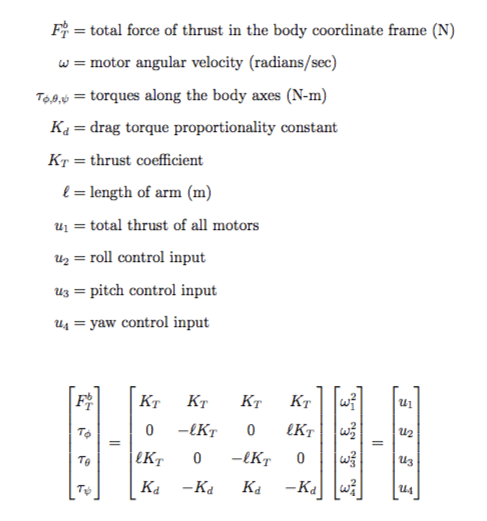Our general control strategy will rely on 3 control subsystems. The sensors on-board the quadrotor measure the angular velocity and translational acceleration. These values are compared to the desired attitude and positions to calculate an error signal that is fed into the control systems. In the first subsystem, a position error is the input and desired roll and pitch angles are the outputs. Next, the roll, pitch, and yaw errors are input into an attitude subsystem. This system outputs desired angular velocities for each axis. These are fed into the final control system. The outputs of this system are the control inputs that can then be mapped into desired motor speeds. This general strategy can be used to develop control systems of varying levels of complexity.

###### Simplified Equations of Motion:

The complex model of the quadrotor’s motion make it difficult to gain accurate insight into the quadrotor’s behavior and to troubleshoot control systems in simulation. For this research, only the primary elements that describe the quadrotor’s behavior at hover, our desired operational state, are considered. The elements that significantly impact the quadrotor’s behavior at high speeds are ignored. We can make several assumptions that reduces the complexity of the non-linear equations described above to develop basic control strategies. We can then increase the complexity of the system model to develop more precise control systems in the future. The model derived in the System Modeling section is reproduced below with the control inputs introduced.The primary assumption made to simplify the model is that the quadrotor will be operated around a stable hover with small attitude angles and minimal rotational and translational velocities and accelerations. Since there are no aerodynamic lifting surfaces, we will assume that the aerodynamic forces and moments are negligible. The effects that are considered negligible are treated as disturbances in the control system and can be compensated for with appropriate control system design. These assumptions are described mathematically as well as the resulting simplified equations of motion. The equations below continue to reduce in complexity as more assumptions are made.While these equations simplify the control design and analysis process, we can still use the more accurate, non-linear equations of motion in the simulated environment to assess the robustness of our control system. Note that in the final equations, translational movement has been decoupled from attitude assuming the heading remains around 0 degrees. Movement along the global x and y axis can be controlled by independently changing the roll and pitch values. Also, for the quadrotor to maintain a stable altitude, the net thrust vector divided by the quadrotor’s mass must be equal to the acceleration due to gravity. The figures below compare the simplified model derived above with the complex model and ground truth measurements described in the Model Verification section. While note as accurate as the non-linear model, the linearized model does compare well to the ground truth. The assumptions needed to linearize the model are validated and the model can be used as a basis for control system design.

###### Simplified PID Control

The most common type of control system is the Proportional-Integral-Derivative (PID) control system. The PID controller is known as a closed loop feedback system. The controller calculates the difference between the actual and desired state and produces an error value. The measurements from the quadrotor’s sensors are fed back to compute this error signal, closing the loop from output to input. This controller is popular because it is somewhat intuitive to tune, easy to implement in code with little processing power, and utilizes measurements that are available on-board most systems. The output of any PID control system is a control value u that will drive the system closer to the desired state. The composition of the PID controller is shown below in both the time domain and frequency domain.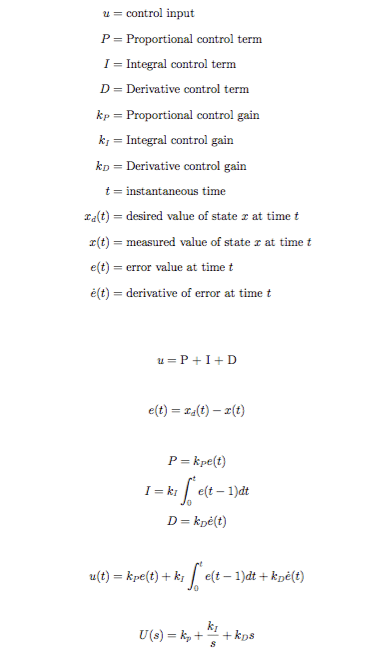The proportional term is any number greater than 0 and results in a value that is just some multiple of the error signal. The integral term is a multiple of the sum of the error accumulated over time. This term responds to a build-up any remaining steady-state error. This error often represents a disturbance (wind, off center payload, modeling errors, etc) that couldn’t be accounted for by the proportional or derivative terms. Therefore, the integral term is used to make small, final adjustments to reduce the final steady-state error. The derivative term is a multiple of the rate of change of the error. This term dampens the response of the control system and is used to reduce overshoot of the system beyond the desired state. A block diagram representation of the basic PID controller is shown below.There are various methods that can be used to tune the PID controller and determine the best values of the control gains given specific system response parameters. For this project, the values were first determined in simulation and then refined on the actual system. Note that for manual RC flight of the quadrotor, only the attitude controller is necessary. The position controller is only needed when using an external localization sensor such as a GPS and conducting waypoint navigation. Note that there are some characteristics of the physical system that a basic PID controller may not accurately account. These include delays in sensor measurements and motor response as well as the inertia of the vehicle.

###### Nested Control Loops

Quadrotor control is often implemented using nested control loops. The inner most loop controls the angular velocities of each axis of the quadrotor. This loop needs to run at a high frequency due to the fast dynamics of the quadrotor. The next highest loop controls the attitude and altitude of the quadrotor. Small attitude changes are directly related to translational acceleration. This means small attitude errors can cause large and unwanted translational displacements very quickly. One benefit to running the rate control loop at a high frequency is that the onboard sensors such as the accelerometer and gyroscope also run at a high frequency. This means we can take advantage of the most recent and accurate measurements when calculating control values. This altitude/attitude controller can receive desired altitude and attitude from a remote controller or from a translational control outer loop.

The outer loop controls translational movement along the x and y axis. This controller outputs desired roll and pitch angles for the inner controller based on the error in the x and y axis. There are multiple inputs for this outer loop controller. These could be waypoints generated from a Ground Control Station, a path planning algorithm, or a visual servoing system for example. A block diagram demonstrating these nested control loops and the quadrotor plant system is shown below.###### Position Control

While the attitude/altitude controller can maintain a stable hover for the quadrotor and track desired attitude/altitude inputs from a RC or GCS, it is unable to control the translational motion of the quadrotor. No matter how accurate the attitude controller may be, small attitude angles will result in translational movement or drifting of the quadrotor. In order to prevent this translational drifting behavior, a position controller is implemented. In order for this controller to function, some sort of relative or global localization must be done. Typically this is done with a camera for body frame position (visual servoing) or a GPS for global frame positioning. This outer loop can run at a slower speed compared to the inner loop controller. The inputs to the position controller are position measurements and the outputs are desired roll and pitch angles to maneuver the quadrotor to the desired x and y position. This controller also enables trajectory following where a series of successive waypoints can be fed to the position controller to get the quadrotor to follow a specific three dimensional path.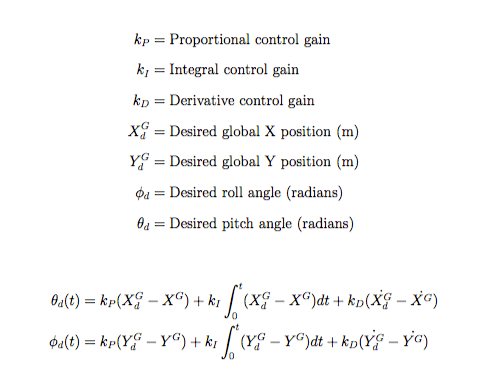###### Attitude and Altitude Control

This section details the development of the attitude and altitude control system. Initially, we want to implement this controller to stabilize the quadrotor around a stable hover. This means that all desired angles are 0 and we set the desired altitude to some low value for tuning purposes. Later, these desired attitude and altitude values can vary based on RC or outer loop controller inputs. Since our simplified model removed any cross coupling in the equations of motion, attitude control can be implemented with an independent control input for each Euler angle.

Controlling the altitude is analogous to controlling the total thrust of the quadrotor. This is important since this control input also influences movement along the x and y axis. This can be seen in the translational equations of motion where u1 is an input in the x and y directions. Therefore, higher values of throttle result in more aggressive movements in the x and y directions given constant roll and pitch angles. In our simplified model, vertical acceleration is decoupled from attitude angle. However, this doesn’t hold when the quadrotor rotates since components of vertical acceleration are also in the x and y planes. Therefore, the altitude control compensates for both the offset of gravity and the deflection of the generalized thrust vector due to tilt. The full PID controllers are detailed below.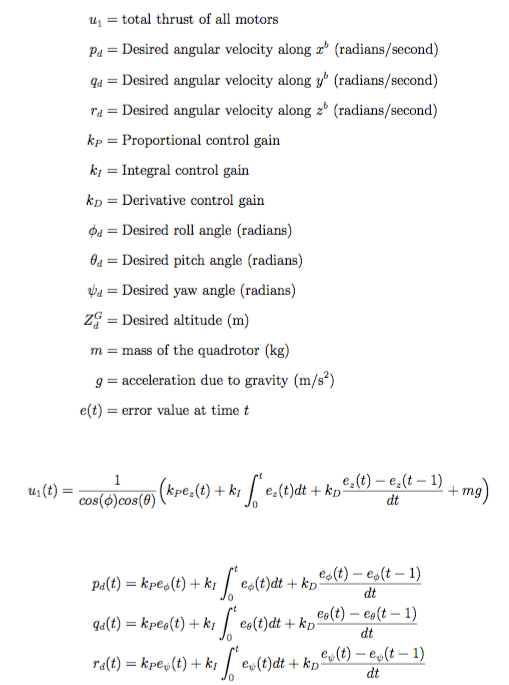Note that yaw/heading control is often accomplished sufficiently with only PD control so the integrator gain would be 0. The code for implementing these control algorithms in discrete time in addition to some modifications to improve performance from the basic PID format are shown in the Autopilot Implementation Section.

###### Angular Velocity Control

The rate controller is the lowest level controller developed for the quadrotor system. This controller receives desired angular rates from the attitude controller. Then, it computes the error between the desired rates and the rates measured by the gyroscope. This error is then used to compute the three moment control inputs. These are combined with the thrust control input calculated by the altitude controller which are then converted to desired motor speeds and sent to the motors via the ESCs.###### Motor Control

Given the control inputs, it is possible to compute the individual motor speeds required for each motor. These speeds will be sent to the ESC which will then send commands to the attached motor to make it spin at the desired speed to produce the desired thrust and control moments. The desired motor speeds can be computed by taking the inverse of the control matrix produced in the section above as shown below. Note that all of the motors contain some component of thrust and yaw control inputs plus the appropriate inputs for each axis. The motors controlling the pitching moment include components of the pitch control input and the motors controlling the rolling moment include components of the rolling control input. These equations are for a “+” quadrotor orientation and would need to be modified if the quadrotor was to be flown in the “X” configuration.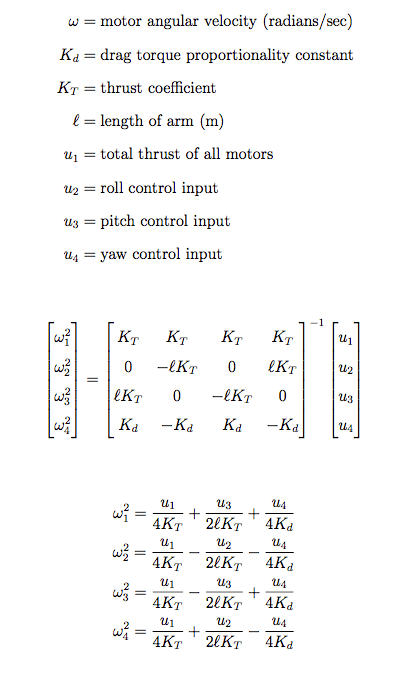It is also important to place limits on the control inputs that can be calculated by the control system. These control inputs are converted into motor speeds that are then sent to the ESCs and eventually the motors. Therefore, we don’t want to use control gains that result in desired motor speeds that are not possible on the actual system. The computations of the control limits are depicted below based on the physical system.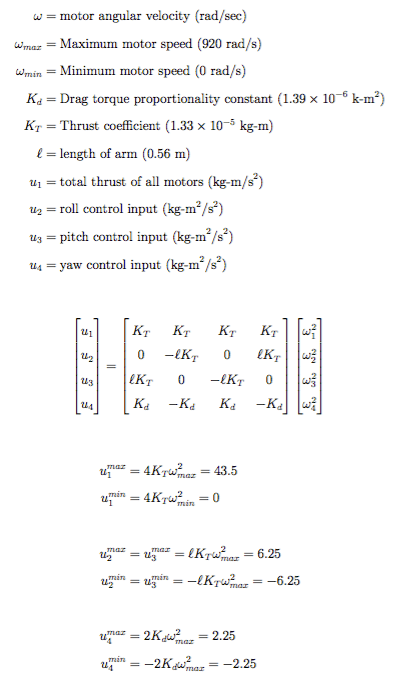###### Basic PID Modifications – Derivative Kick

This section describes a few modifications that can be made to the basic PID controller described above in order to improve flight performance and stability. Brett Beauregard has a more detailed explanations of these modifications on his website.

The first modification minimizes what is known as “derivative kick.” The error is calculated as the difference between the setpoint and current measurement input. Therefore, the derivative of the error is equal to the difference between the derivative of the setpoint and the derivative of the measured input. This means that any change in the setpoint results in a large spike in its derivative. For most applications, the setpoint remains relatively constant. When following a desired trajectory, the setpoint may continue to vary. Regardless, these instantaneous changes in setpoint will result in large derivatives that will be fed into the PID controller. Given the highly nonlinear dynamics of the quadrotor, this could result in instability. Instead, we assume that the derivative of the setpoint doesn’t change often and set its derivative to 0. This results in the derivative of the error being equal to the negative of the derivative of the measured input. The derivative value is often able to be measured with an onboard sensor. The modification results in the following block diagram implementation and the code is shown in the Autopilot Implementation Section. Note that the value of Kd, the derivative gain, is of the opposite sign of the Kd used in the traditional PID implementation.###### Basic PID Modifications – Anti-Windup

The second modification improves stability by placing limits on the integral control term. As discussed previously, the integral term computes the sum of the error over time. The purpose of this term is to compensate for any constant disturbances such as the environment or modeling errors. This term has the most impact when the system has already reached a steady state but has a constant error. The integral term will increase the control output to slowly decrease the steady state error. However, if there is a large error due to a disturbance or sudden change in the setpoint, the integral term can quickly become a large value as it continues to add while the system approaches the desired setpoint. Even once the system gets near the desired value, the integral term is still very large and can force the system to overshoot, become unstable, or to oscillate. Additionally, due to the large integral build-up, if the setpoint changes in the opposite direction, the integral term will delay the controller’s response as it waits for the integral value to decrease. To fix this, we only enable the integral term once we are in a region around the steady state that is controlled. This lets the integral term eliminate any steady state error in a stable fashion. Also, we can saturate the controller’s output to match the minimum and maximum values of the motor input.

[simple-social-share]

## 3 thoughts on “Control Systems Design”

1.yasmine says:

hello,
i try to simulate the drone but there are a problem , the drone don’t change her position could you please help me or give me an idea , thank you

2.carlos says:

Hello wilselby, how are you?
Thank you so much for sharing this good work, it’s hard to find a material explained in this way. By chance you will have the file (if you can share it) with which you get the first graphs comparing the linear model with the non-linear one. I would like to replicate that analysis and observe the results.
I hope you can help me,
thank you very much

3.OMAR MECHALI says:

Thank you for the excellent work, it really helps a lot.
Sir I would like to ask you about the Angular Velocity Control system. In many papers, they just design the position controller and the Attitude and Altitude Control. I’m confused.
Thanks.
Best Regards